# Using PowerShell to Export CSV Files to XML: Part 1

Like many of you, I store a lot of information in CSV files. Although CSV files are easy to use, they are not without limitations. For example, it’s impossible to capture any type of nested data with CSV files. The CSV format is essentially flat, which fails when you try to export or serialize something in PowerShell that has nested objects. When you import a CSV file, another limitation is that everything is treated as a string. With these two issues in mind, I’m going to start a project of building a PowerShell toolset to help move my CSV files to XML.

`​`\$path = "c:\scripts\users.csv"``

I love how easy it is to import a CSV file and turn each line into an object.

In this particular case, I can use this file to create new accounts in Active Directory. Everything’s pretty simple, and I can easily export the data to XML using the Export-Clixml cmdlet.

`​`Import-CSV -Path \$path | export-clixml D:\temp\users.xml``

Although the resulting XML isn’t very pretty, it is intended to be used within PowerShell.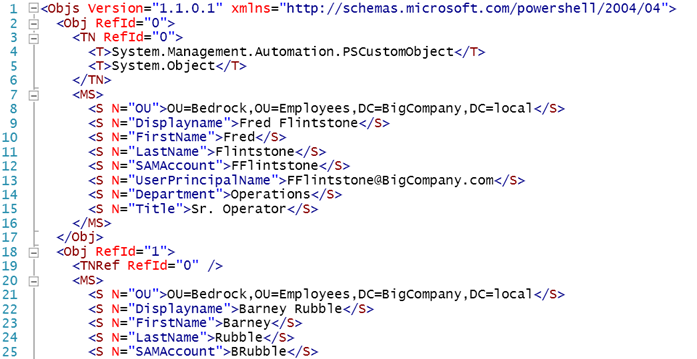Our resulting XML to be used in PowerShell. (Image Credit: Jeff Hicks)

``import-clixml D:\temp\users.xml | select -first 1``

The result is the same.
It might be hard to tell from the XML code, but every item is a string. To make this more challenging, let’s look at a CSV file with potentially different types of data.

If I imported this file with Import-CSV, then everything would be a string, which would make it more difficult to filter or sort. One approach is to add type information on the fly during the import.

```\$data = Import-csv c:\scripts\TestData.csv |
Select @{Name="Date";Expression = {\$_.date -as [datetime]}},
Name,Service,
@{Name="Key";Expression={\$_.Key -as [int]}},
@{Name="Size";Expression={\$_.Size -as [int]}},
@{Name="Test";Expression={[convert]::ToBoolean(\$_.Test)}}```

I am explicitly casting each new property as a certain type. Those that I ignore will be treated as strings.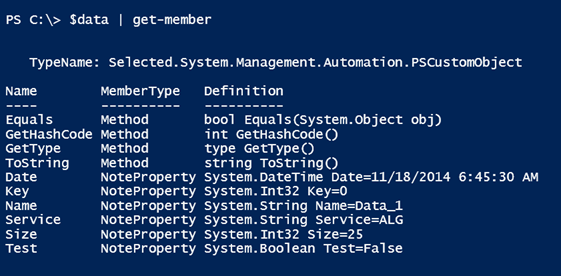This could be exported.

`​`\$data | export-clixml -Path d:\temp\testdata.xml``

And re-importing verifies that type is maintained.

Did you notice I had to do something different for the Boolean value? That’s because the –AS operator can’t handle everything, especially when starting as a string.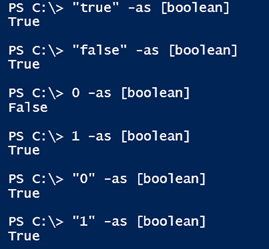However, the [Convert] .NET class seems to do the trick.
Because I’m always looking for easier ways to do things in PowerShell, I had the thought to use a mapping table to define an object type for each imported property.

```\$map = [ordered]@{
Date = [datetime]
Name = [string]
Service = [string]
Key = [int]
Size = [int]
Test = [boolean]
}```

Using an ordered hashtable means everything will be in the specified order. To use this map, I can import my CSV and process each line.

```\$imported = Import-CSV -Path c:\scripts\testdata.csv | foreach {
\$import = \$_
\$map.GetEnumerator() | ForEach -begin {
\$hash = [ordered]@{}
} -process {
} -end {
New-Object -TypeName PSObject -Property \$hash
}
} #foreach```

For each imported object, I’m creating a new ordered hashtable and adding the mapping key and corresponding imported value, properly typed. After I’ve processed the map for each imported object, a new custom object is written to the pipeline.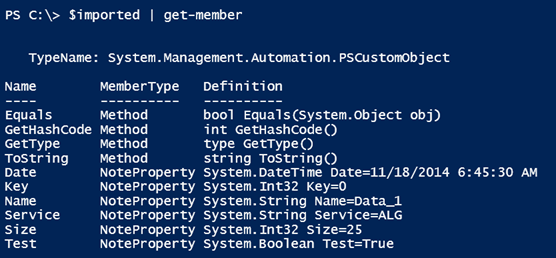A new custom object is being written to the pipeline. (Image Credit: Jeff Hicks)
I still have my Boolean issue. If my CSV file didn’t Boolean values, then this isn’t too bad an approach. With that said, I want something that works in all cases. Eventually, I settled on using a switch construct. My mapping table is slightly different now.

```\$map = [ordered]@{
Date = "datetime"
Name = "string"
Service = "string"
Key = "string"
Size = "int32"
Test = "boolean"
}
```

To use this table, I can use the same concept as before, except

```\$imported = Import-CSV -Path c:\scripts\testdata.csv | foreach {
\$import = \$_
\$map.GetEnumerator() | ForEach -begin {
\$hash = [ordered]@{}
} -process {
\$property = \$_.key
#write-host "\$property = \$(\$import.\$property)" -fore Cyan
#only process if there is a value
if (\$import.\$property) {
Switch (\$_.Value) {
"datetime" { \$value = [convert]::ToDateTime(\$import.\$property) }
"string"   { \$value = [convert]::ToString(\$import.\$property) }
"int32"    { \$value = [convert]::ToInt32(\$import.\$property) }
"int64"    { \$value = [convert]::ToInt64(\$import.\$property) }
"boolean"  { \$value = [convert]::ToBoolean(\$import.\$property) }
"double"   { \$value = [convert]::ToDouble(\$import.\$property) }
Default    { \$value = \$import.\$property }
} #switch
} #if there is a property value
else {
#set the value to null
\$Value = \$Null
}
} -end {
#write a custom object to the pipeline
New-Object -TypeName PSObject -Property \$hash
}
} #foreach
```

As each imported object is created, I create a different object, casting each property to its corresponding type. My switch statement uses what I think are the most common types you are likely to use. If there is no property value, then I expressly set the value to \$Null. You may want different behavior.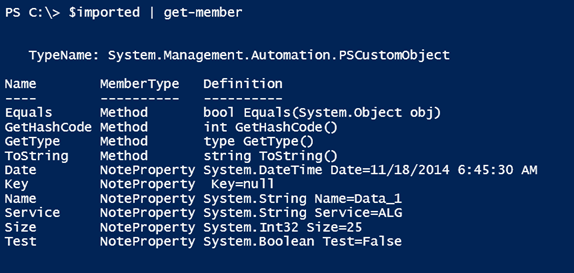With this proof of concept code, I can create a re-usable function.

```Function Convert-MyCSV {
[cmdletbinding()]
Param(
[Parameter(Position=0,ValueFromPipeline)]
[object]\$Inputobject,
[Parameter(Mandatory,HelpMessage = "Enter an ordered hashtable of property names and types")]
[Alias("map")]
[System.Collections.Specialized.OrderedDictionary]\$PropertyMap
)
Begin {
Write-Verbose "Starting \$(\$MyInvocation.Mycommand)"
Write-Verbose "Using property map \$(\$PropertyMap | out-string)"
} #begin
Process {
\$PropertyMap.GetEnumerator() | ForEach -begin {
\$hash = [ordered]@{}
} -process {
\$property = \$_.key
write-Verbose "\$property = \$(\$InputObject.\$property)"
#only process if there is a value
if (\$InputObject.\$property) {
Switch (\$_.Value) {
"datetime" { \$value = [convert]::ToDateTime(\$InputObject.\$property) }
"string"   { \$value = [convert]::ToString(\$InputObject.\$property) }
"int32"    { \$value = [convert]::ToInt32(\$InputObject.\$property) }
"int64"    { \$value = [convert]::ToInt64(\$InputObject.\$property) }
"boolean"  { \$value = [convert]::ToBoolean(\$InputObject.\$property) }
"double"   { \$value = [convert]::ToDouble(\$InputObject.\$property) }
Default    { \$value = \$InputObject.\$property }
} #switch
} #if there is a property value
else {
#set the value to null
\$Value = \$Null
}
} -end {
#write a custom object to the pipeline
New-Object -TypeName PSObject -Property \$hash
}
} #process
End {
Write-Verbose "Ending \$(\$MyInvocation.Mycommand)"
} #end
} #end function
```

The intention is that you would run Import-CSV and pipe to Convert-MyCSV. My function requires a mapping hash table, so I put together a function to help generate it.

```Function New-CSVMap {
[cmdletbinding()]
Param(
[Parameter(Position=0,Mandatory,HelpMessage="Enter the path to the CSV file")]
[ValidateScript({
if (Test-Path \$_) {
\$True
}
else {
Throw "Cannot validate path \$_"
}
})]
[string]\$Path,
[ValidateNotNullorEmpty()]
[string]\$Delimiter = ",",
[ValidateSet("ASCII","Unicode","UTF7","UTF8","UTF32","BigEndianUnicode","Default","OEM")]
[string]\$Encoding = "ASCII"
)
#temporarily import data
\$temp = Import-CSV -Path \$path -Delimiter \$Delimiter -Encoding \$encoding
#initialize an ordered hashtable
\$map=[ordered]@{}
} -process {
#strip off any quotes
\$property = \$_.replace('"',"")
#get sample value from the first imported object that has a value
\$sample = \$temp.where({\$_.\$property})| Select -first 1 -ExpandProperty \$Property
#prompt for object type
\$type = Read-Host "What type is \$property [ \$sample ]"
} -end {
#Write the result to the pipeline
\$map
}
} #end function
```

The function assumes this is a typical CSV file with the header as the first line. It then imports some sample data and prompts you to specify an object type.

Armed with this, I can easily import the CSV and end up with typed data.

`​`\$data = Import-csv c:\scripts\mydata.csv | Convert-MyCSV -PropertyMap \$map``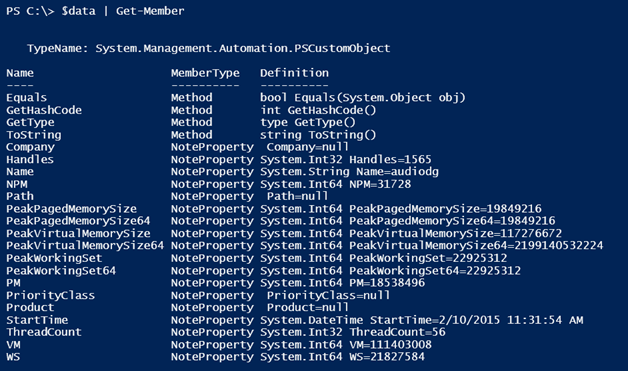Importing the CSV file to obtain typed data. (Image Credit: Jeff Hicks)
As an alternative, you could do everything in one line:

`​`Import-csv c:\scripts\testdata.csv | Convert-MyCSV -PropertyMap (New-csvMap c:\scripts\testdata.csv)``

In any event, the end result is proper data that I could export to XML. Or do it all in one fell swoop.

`​`Import-csv c:\scripts\testdata.csv | Convert-MyCSV -PropertyMap (New-csvMap c:\scripts\testdata.csv) | export-clixml c:\scripts\testdata.xml``

But if I want to use the XML outside of PowerShell, that might be a bit more complicated. We’ll look at that in another article.